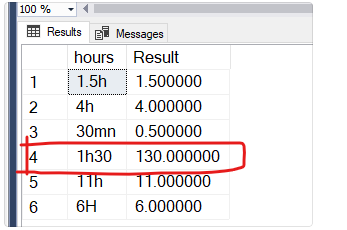# Want to convert time field into hour format

I have included all the possibility that hours could come in our production DB. The result column is generated from an hour column, where every time is converted into hour format. My code is not working for the 4th case(I marked with red). Any help with how I can fix this. Till Now, I have tried this sql thing.

``````SELECT hours,result=(CASE
WHEN hours LIKE '%h%' and hours NOT LIKE '%m%'
THEN SUBSTRING(hours,1,CHARINDEX('h', hours)-1) + Right(SUBSTRING(hours,CHARINDEX('h', hours)+1,2),2)
WHEN hours LIKE '%mn%'
THEN CAST(SUBSTRING(hours,1,CHARINDEX('m', hours)-1) as decimal(10,3))/60
ELSE 'FAIL'
END)
FROM aaa_Test_hours
``````
hours result
1.5h 1.500000
4h 4.000000
30mn 0.500000
1h30 130.000000
11h 11.000000
6H 6.000000What does the hours column indicate?

hours column means how many hours taken to complete the particular task. For example:line 4 "1h30" means 1 hour 30 minutes to complete this task. as you see in result column is showing 130 hours that is totally wrong. The correct should value should be 1.500000. hours datatype is varchar.

look at 1.5h what does that mean vs 1h30?
highly recommend you change this unless this is your effort to do that?

use a real time field or something other than varchar

hope this helps

``````declare @hours varchar(10) = '1h30'

select
cast(SUBSTRING ( @hours ,0 , charindex('h',@hours) )  as int ) +    cast(SUBSTRING ( @hours ,charindex('h',@hours)+1 , 2 )  as int)/60.0
``````

``````DROP TABLE IF EXISTS #aaa_Test_hours
CREATE TABLE #aaa_Test_hours ( hours varchar(40) NULL );
INSERT INTO #aaa_Test_hours VALUES('1.5h'),('4h'),('30mn'),('1hr30'),('11h'),('6h'),
('20m4')

SELECT
hours,
CASE WHEN second_value IS NULL
THEN first_numeric / CASE WHEN first_type = 'h' THEN 1.0 ELSE 60.0 END
WHEN second_value = -1
THEN NULL
WHEN first_type = 'm'
THEN first_numeric / 60.0 + second_numeric
ELSE first_numeric + second_numeric / 60.0
END AS result,
CASE WHEN second_value = -1 THEN 'FAILED' ELSE '' END AS message
FROM #aaa_Test_hours
CROSS APPLY (
SELECT PATINDEX('%[^0123456789.]%', hours) AS first_nonnumeric_byte
) AS ca1
CROSS APPLY (
SELECT CAST(LEFT(hours, first_nonnumeric_byte - 1) AS decimal(6, 2)) AS first_numeric,
SUBSTRING(hours, first_nonnumeric_byte, 1) AS first_type
) AS ca2
CROSS APPLY (
SELECT first_nonnumeric_byte + NULLIF(PATINDEX('%[0123456789.]%',
SUBSTRING(hours, first_nonnumeric_byte + 1, 200)), 0) AS second_numeric_byte
) AS ca3
CROSS APPLY (
SELECT SUBSTRING(hours, second_numeric_byte, 20) AS second_value
) AS ca4
CROSS APPLY (
SELECT CASE WHEN second_value LIKE '%[^0123456789.]%' OR
second_value LIKE '%.%.%' THEN -1
ELSE CAST(second_value AS decimal(6, 2)) END AS second_numeric
) AS ca5``````

the column is varchar so this will blow up once someone enters ('mickey.mouse')

What would happen if someone enters 30m - or '1 hour 20 minutes' - or someone decides to get cute and enter 30m1h?

The source column really needs to be a duration instead of an external 'time'.

1 Like

If that actually becomes an issue, best would be a trigger on the table that would prevent bad data from being inserted in the first place.

And whatever app is populating this database needs a simple non free text entry

Drop down with

``````Hour     minutes
1            30
``````